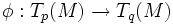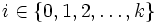# Curvature-homogeneous pseudo-Riemannian manifold

A pseudo-Riemannian manifold$(M,g)$ is said to be curvature-homogeneous up to order$k$ if for any points$p, q \in M$ there exists a linear isometry$\phi:T_p(M) \to T_q(M)$ such that for all integers$i \in \{ 0,1,2, \ldots, k \}$ the following holds:$\phi^k (\nabla^i R(p)) = \nabla^i R(q)$
where$\nabla$ denotes the covariant derivative with respect to the Levi-Civita connection and$R$ denotes the Riemann curvature tensor.